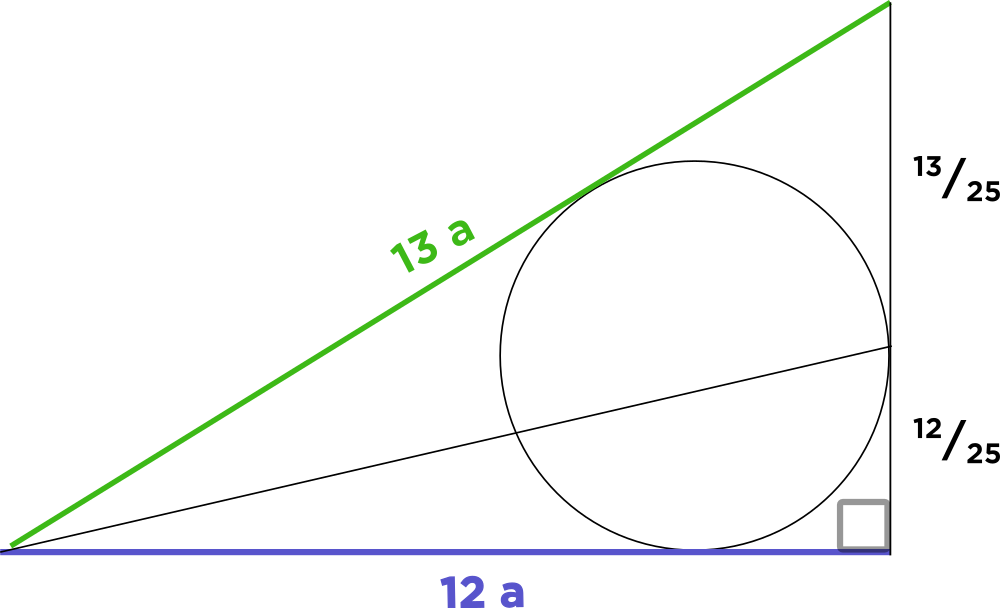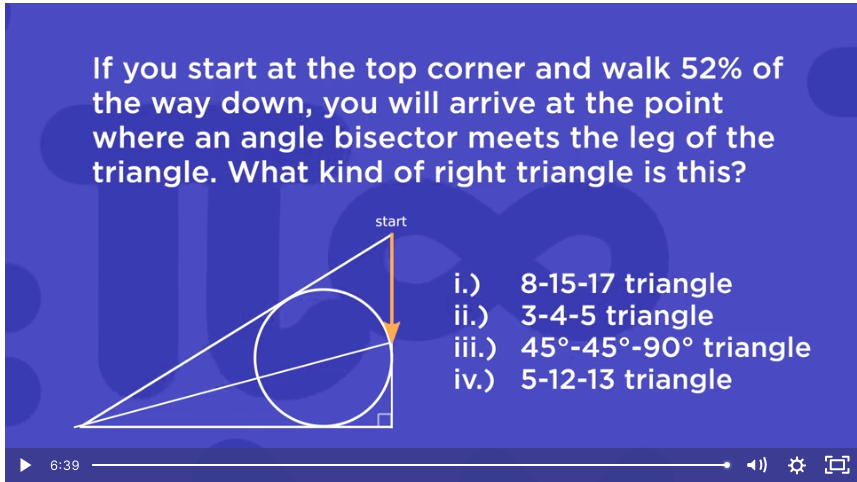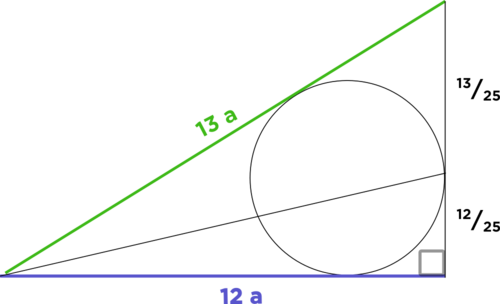# QUESTION!? Where did we get 13a and 12a?

• Module 2 Week 3 Day 12 Your Turn Part 2

I THOUGHT PO SHEN LOH SAID "ANY OLD 30-60-90" RIGHT TRIANGLE. SO WHY IS THE ANSWER TO TO DAY 13 YOUR TURN PART 2 A TRIANGLE THAT ISN'T A 30 60 90. SOMEHOW IT'S A 5 12 13. WOW MAGIC! HOPE YOU HEAR THE SARCASM!!!

• @bravekiwi Yes, you are right that at the very end, Prof. Loh says, "This question shows us what happens when you take any old 30-60-90 right triangle and draw a line though the center." Even though the mini-question at the end of the Your Turn (Part 2) video seems like a very different triangle, it's actually illustrating the same concept as in the original Your Turn question! We could even use a random triangle to illustrate this concept, which has a nice name: it's called the Angle Bisector Theorem.

When you have a line bisecting an angle in a triangle, that line will cut the opposite side in two parts, the ratios of which are in the same ratio as the other two sides of the triangle!So the ratio of the green and purple sides, $$13:12,$$ is the same as the ratio of the segments cut from the third side, $$13:12.$$

In fact, if we had used another $$30^{\circ}-60^{\circ}-90^{\circ}$$ triangle, then it might not have been obvious that this fact works for any triangle! It might have appeared to be a property of only $$30^{\circ}-60^{\circ}-90^{\circ}$$ right triangles.• Where did we get 13a and 12a?

• @TSS-Graviser Hi there, the $$13a$$ and $$12a$$ come from the fact that we know the ratios of the $$\textcolor{green}{\text{green}}$$ and $$\textcolor{blue}{\text{blue}}$$ sides. How do we know the ratio of the $$\textcolor{green}{\text{green}}$$ and $$\textcolor{blue}{\text{blue}}$$ sides? From the information given in the question, that the yellow segment is 52% of the vertical side.Since $$52%$$ equals the fraction $$\frac{52}{100},$$ which equals $$\frac{4 \times 13}{4 \times 25},$$ which reduces to $$\frac{13}{25},$$ this means that the other piece of the vertical line under the yellow arrow is $$1 - \frac{13}{25} = \frac{12}{25}$$ of the whole vertical side.

That's how we got this picture here:Do you see that Angle Bisector Theorem here tells us that the ratio of the $$\textcolor{green}{\text{green}}$$ and $$\textcolor{blue}{\text{blue}}$$ sides is $$13 : 12$$ ?

We can't say for sure that the $$\textcolor{green}{\text{green}}$$ side is $$13$$ long and the $$\textcolor{blue}{\text{blue}}$$ side is $$12$$ long, but we just know that the length of the $$\textcolor{green}{\text{green}}$$ side divided by the length of the $$\textcolor{blue}{\text{blue}}$$ side is equal to $$\frac{13}{12},$$ so we say that the $$\textcolor{green}{\text{green}}$$ side has length $$13$$ units of some amount, which is $$13a,$$ and the $$\textcolor{blue}{\text{blue}}$$ side has length $$12$$ units of some amount, which is $$12a.$$